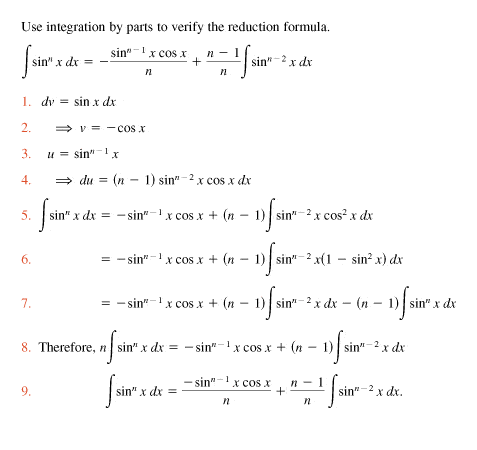# Integration By Parts Problem

## Homework Statement## The Attempt at a Solution

I tried this problem and couldn't figure it out so I went and got the solution. However, I don't understand step 6 of the solution. I'm not sure how

$$(n-1)\int\sin^{n-2}x(1-\sin^2x)dx=(n-1)\int\sin^{n-2}dx-(n-1)\int\sin^nx dx$$